Ex 11.2

Chapter 11 Class 12 Three Dimensional Geometry
Serial order wise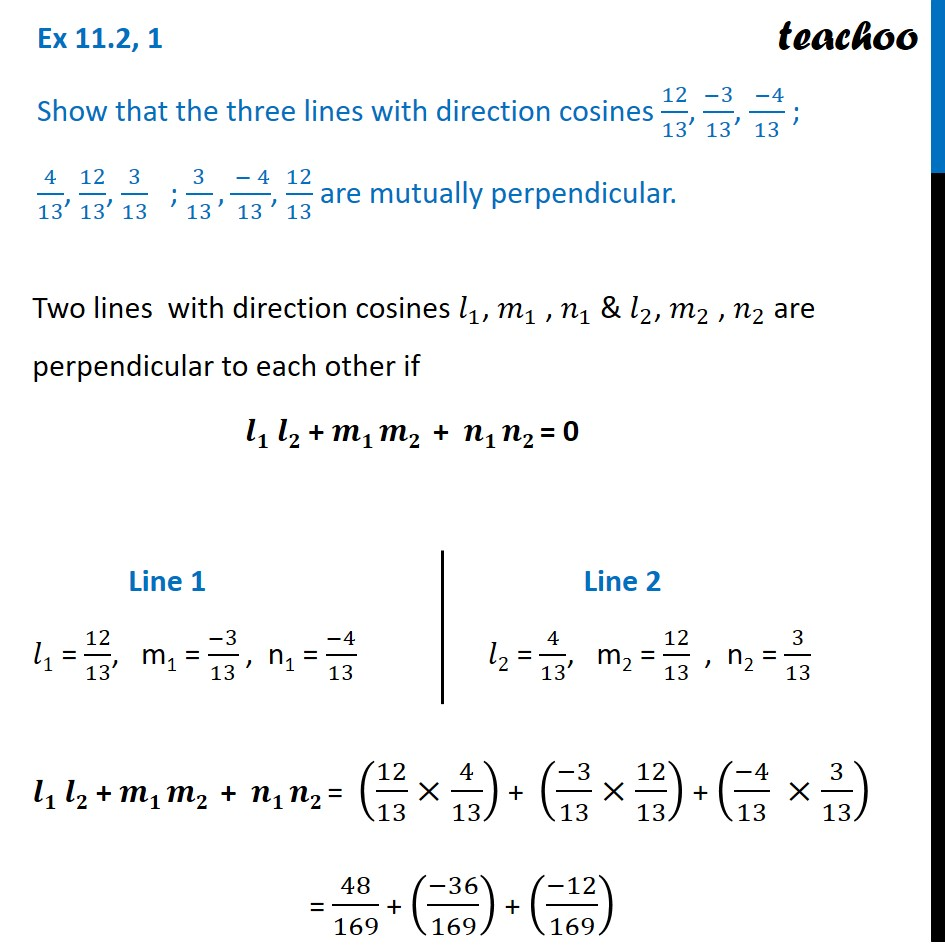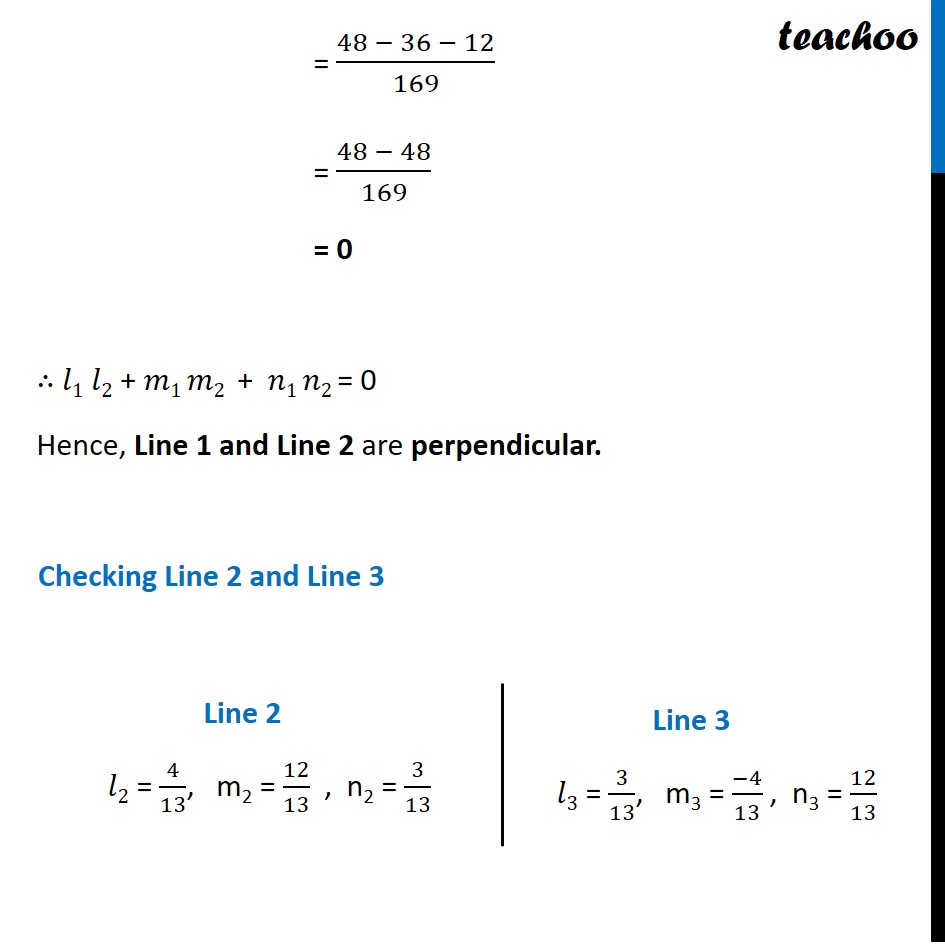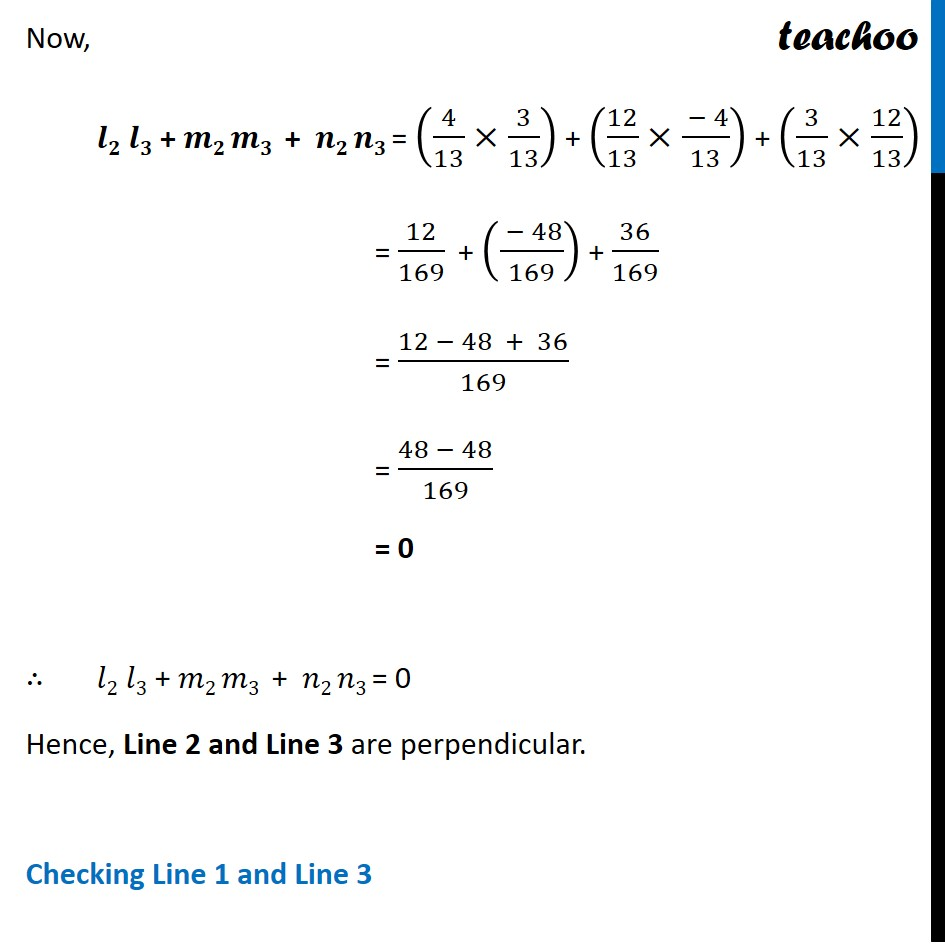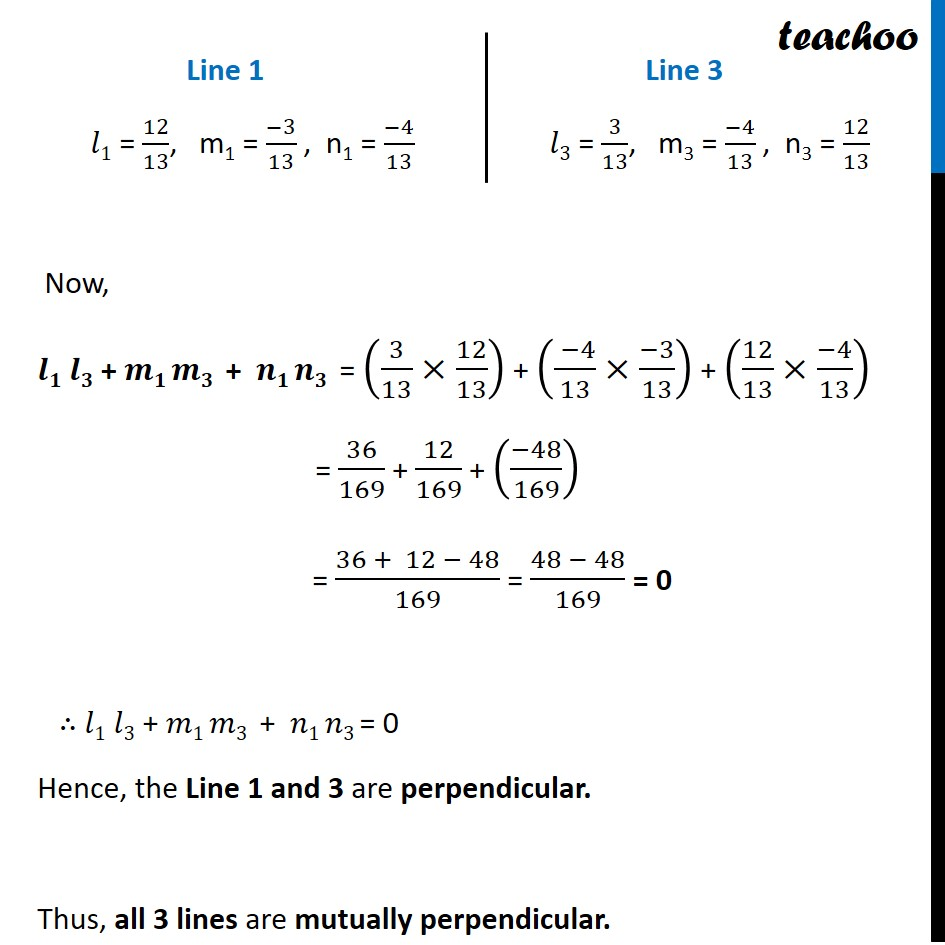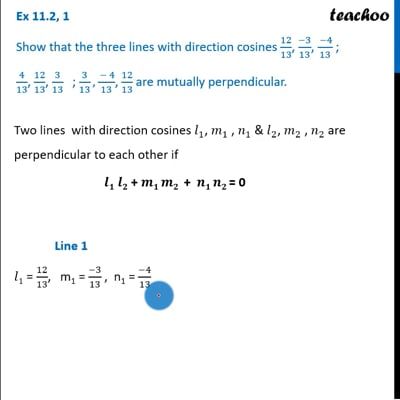This video is only available for Teachoo black users

Solve all your doubts with Teachoo Black (new monthly pack available now!)

### Transcript

Ex 11.2, 1 Show that the three lines with direction cosines 12/13, (−3)/13, ( −4)/13 ; 4/13, 12/13, 3/13 ; 3/13,( − 4)/13, 12/13 are mutually perpendicular. Two lines with direction cosines 𝑙_1, 𝑚_1 , 𝑛_1 & 𝑙_2, 𝑚_2 , 𝑛_2 are perpendicular to each other if 𝒍𝟏 𝒍𝟐 + 𝒎𝟏 𝒎𝟐 + 𝒏𝟏 𝒏𝟐 = 0 𝒍𝟏 𝒍𝟐 + 𝒎𝟏 𝒎𝟐 + 𝒏𝟏 𝒏𝟐 = (12/13×4/13) + ((−3)/13×12/13) + ((−4)/13 ×3/13) = 48/169 + ((−36)/169) + ((−12)/169) Line 1 𝑙1 = 12/13, m1 = (−3)/13 , n1 = (−4)/13 Line 2 𝑙2 = 4/13, m2 = 12/13 , n2 = 3/13 = (48 − 36 − 12)/169 = (48 − 48)/169 = 0 ∴ 𝑙1 𝑙2 + 𝑚1 𝑚2 + 𝑛1 𝑛2 = 0 Hence, Line 1 and Line 2 are perpendicular. Checking Line 2 and Line 3 Line 2 𝑙2 = 4/13, m2 = 12/13 , n2 = 3/13 Line 3 𝑙3 = 3/13, m3 = (−4)/13 , n3 = 12/13 Now, 𝒍𝟐 𝒍𝟑 + 𝒎𝟐 𝒎𝟑 + 𝒏𝟐 𝒏𝟑 = (4/13×3/13) + (12/13×( − 4)/13) + (3/13×12/13) = 12/169 + (( − 48)/169) + 36/169 = (12 − 48 + 36)/169 = (48 − 48)/169 = 0 ∴ 𝑙2 𝑙3 + 𝑚2 𝑚3 + 𝑛2 𝑛3 = 0 Hence, Line 2 and Line 3 are perpendicular. Checking Line 1 and Line 3 Line 1 𝑙1 = 12/13, m1 = (−3)/13 , n1 = (−4)/13 Line 3 𝑙3 = 3/13, m3 = (−4)/13 , n3 = 12/13 Now, 𝒍𝟏 𝒍𝟑 + 𝒎𝟏 𝒎𝟑 + 𝒏𝟏 𝒏𝟑 = (3/13×12/13) + (( −4)/13×(−3)/13) + (12/13×(−4)/13) = 36/169 + 12/169 + ((−48)/169) = (36 + 12 − 48)/169 = (48 − 48)/169 = 0 ∴ 𝑙1 𝑙3 + 𝑚1 𝑚3 + 𝑛1 𝑛3 = 0 Hence, the Line 1 and 3 are perpendicular. Thus, all 3 lines are mutually perpendicular.